# GRE Subject Test: Physics : Circuits

## Example Questions

### Example Question #1 : Circuits

What is the total resistance of a circuit containing four resistors (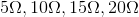) hooked up in series.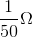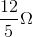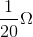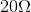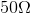Explanation:

For a circuit in series, the total resistance is simply given by the sum of each individual resistor: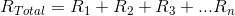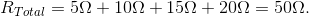### Example Question #2 : Circuits

What is the total resistance of a circuit consisting of three resistors in a parallel configuration? The resistors have the following resistance: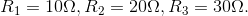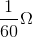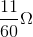Cannot be determined.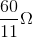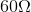Explanation:

The total resistance of a circuit in parallel is given by the following equation: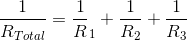Now, we just plug in the values, and solve for the total resistance by inverting!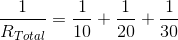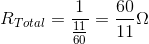### All GRE Subject Test: Physics Resources